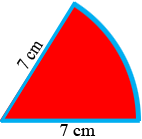SEARCH HOMEMath Central Quandaries & QueriesQuestion from Alisa: find the perimeter of the sector with radius 7 cm in which the angle at the centre is 60 degrees leave your answer in terms of piHi Alisa,

I drew a diagram with the perimeter in blue.What is 360 divided by 60? What is the length of the perimeter?

PennyMath Central is supported by the University of Regina and The Pacific Institute for the Mathematical Sciences.